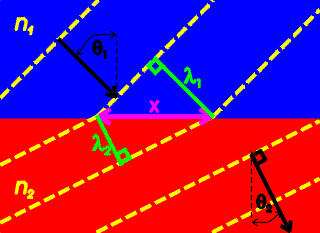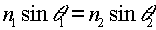Snell's law Light rays that cross a surface to a medium with a different index of refraction will bend. Snell's law relates the angles of incidence and refraction to the ratio of the material's indices of refraction. Bending the light rays via Snell's law is the foundation of designing lenses for telescopes, eyeglasses and microscopes.The image on the right shows light incident from the blue medium at an angle of incidence, q1, that enters the red medium with an angle of refraction, q2. The yellow lines represent the wave fronts. Some geometry shows:From the previous page one knows that the wavelengths are inversely proportional to the indices of refraction.Combining the two equations above gives Snell's law.Thus if light is incident on a surface with incident angle q1, one can find the angle of refraction q2 using Snell's law.